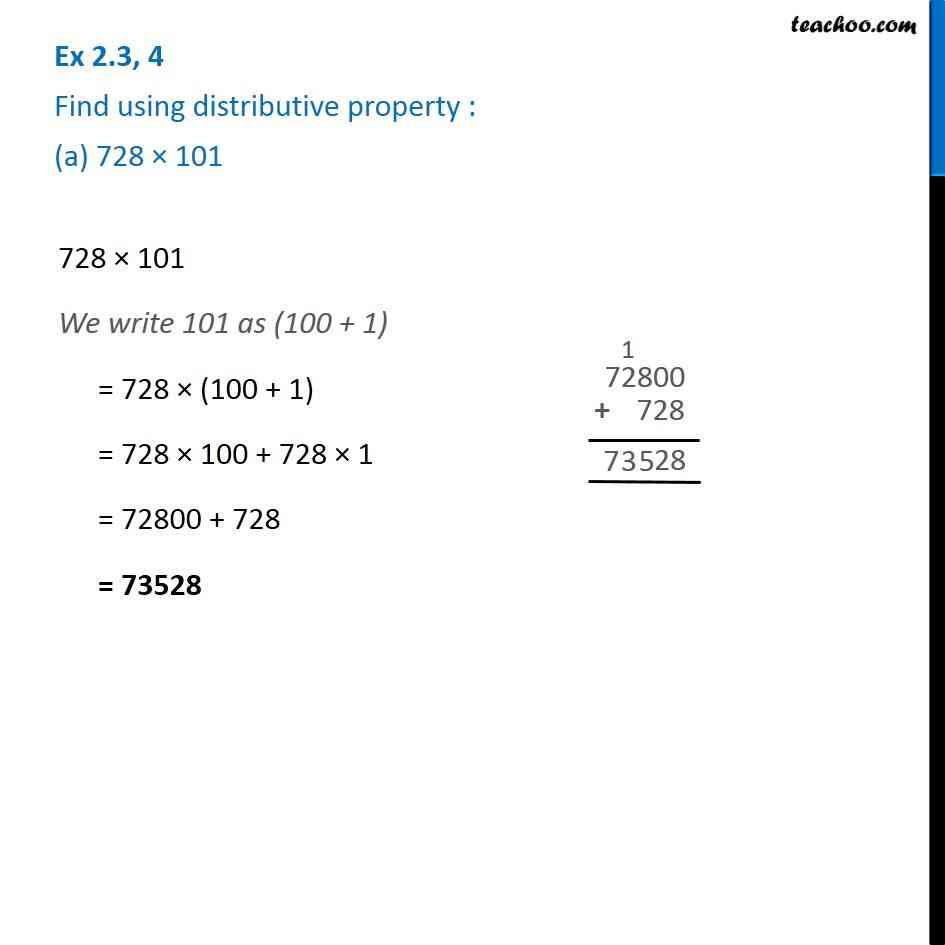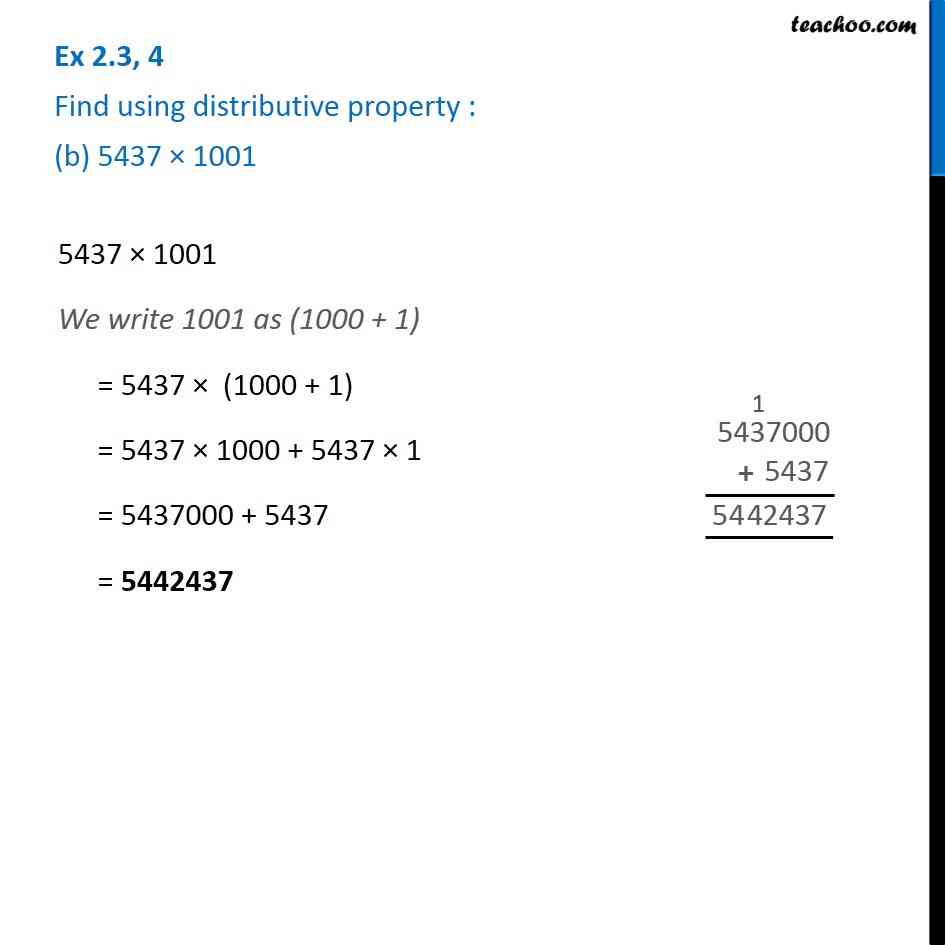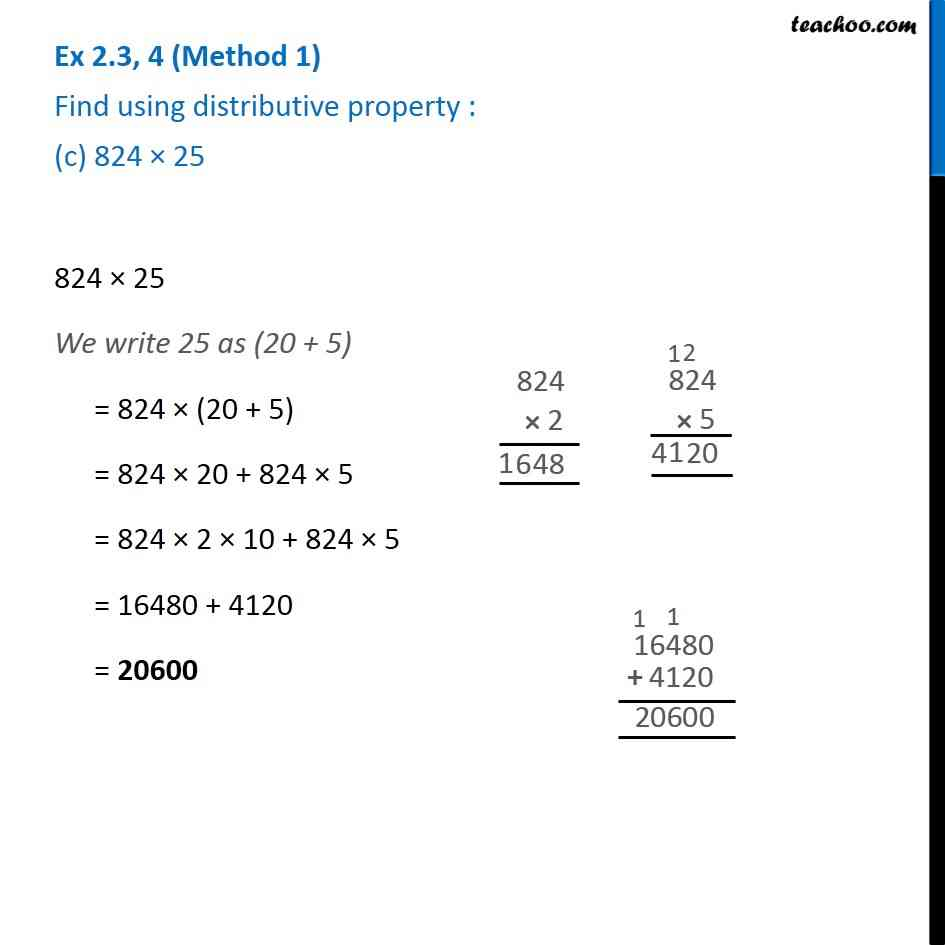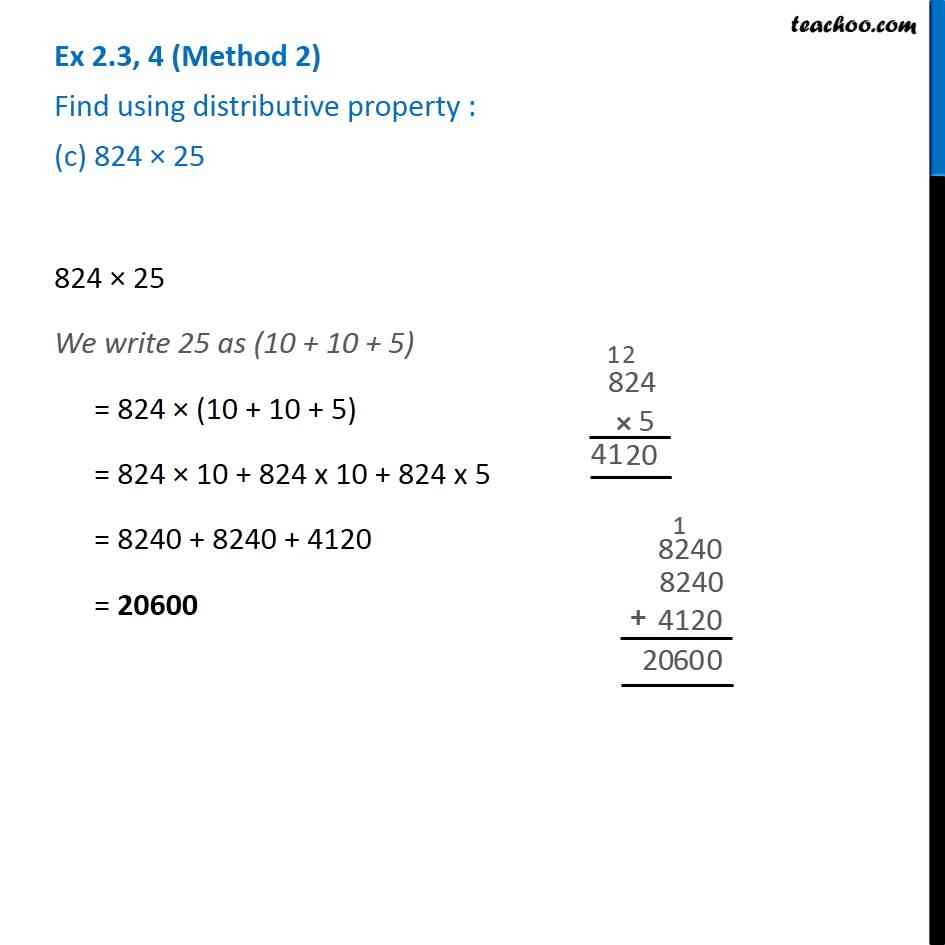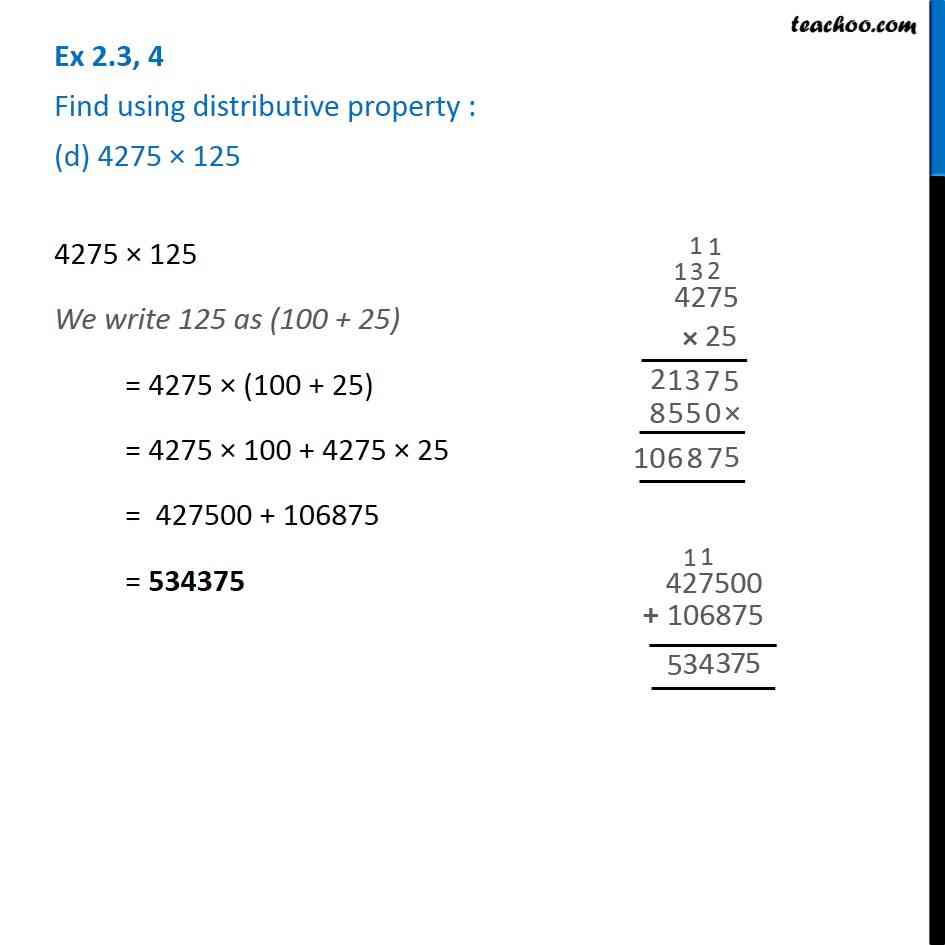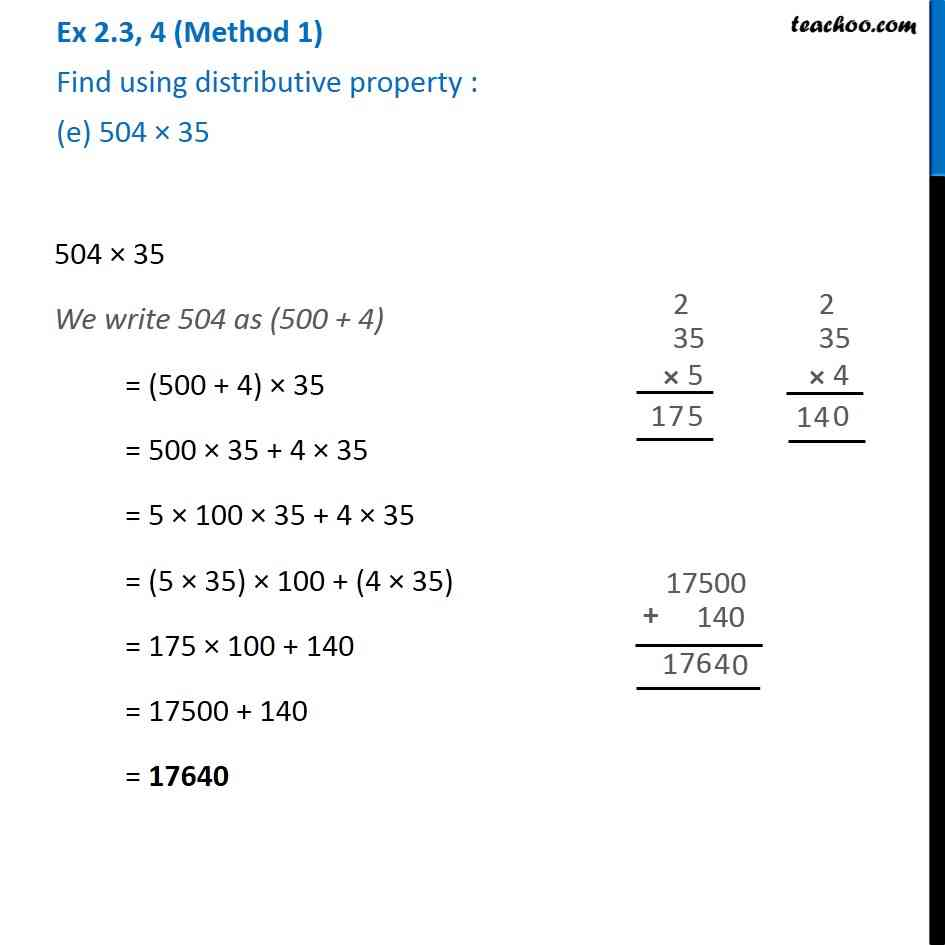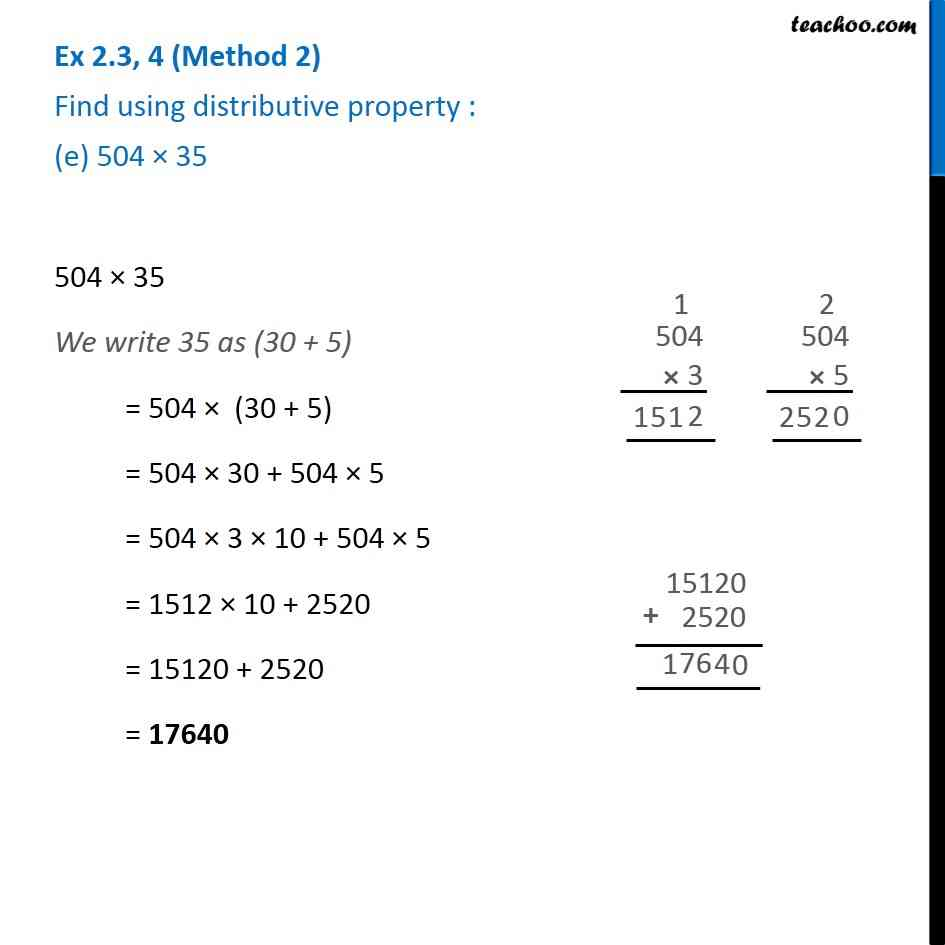Subscribe to our Youtube Channel - https://you.tube/teachoo

1. Chapter 2 Class 6 Whole Numbers
2. Concept wise
3. Distributivity

Transcript

Ex 2.3, 4 Find using distributive property : (a) 728 × 101728 × 101 = 728 × (100 + 1) = 728 × 100 + 728 × 1 = 72800 + 728 = 73528 Ex 2.3, 4 Find using distributive property : (b) 5437 × 1001 5437 × 1001 = 5437 (1000 + 1) = 5437 × 1000 + 5437 × 1 = 5437000 + 5437 = 5442437 Ex 2.3, 4 Find using distributive property : (c) 824 × 25824 × 25 = 824 × (20 + 5) = 824 × 20 + 824 × 5 = 824 × 2 × 10 + 824 × 5 = 16480 + 4120 = 20600 Ex 2.3, 4 (2nd method ) Find using distributive property : (c) 824 × 25824 × 25 = 824 × (10 + 10 + 5) = 824 × 10 + 824 x 10 + 824 x 5 = 8240 + 8240 + 4120 = 20600 Ex 2.3, 4 (2nd method ) Find using distributive property : (c) 824 × 25824 × 25 = 824 × (10 + 10 + 5) = 824 × 10 + 824 x 10 + 824 x 5 = 8240 + 8240 + 4120 = 20600 Ex 2.3, 4 (3rd method ) Find using distributive property : (c) 824 × 25824 × 25 = 824 × 100/4 = 824/4 × 100 = 206 × 100 = 20600 4275 × 125 = 4275 × (100 + 25) = 4275 × 100 + 4275 × 25 = 427500 + 106875 = 534375 Ex 2.3, 4 (Method 1) Find using distributive property : (e) 504 × 35504 × 35 = (500 + 4) × 35 = 500 × 35 + 4 × 35 = 5 × 100 × 35 + 4 × 35 = (5 × 35) × 100 + 4 × 35 = 175 × 100 + 140 = 17500 + 140 = 17640 Ex 2.3, 4 (Method 2) Find using distributive property : (e) 504 × 35504 × 35 = 504 (30 + 5) = 504 × 30 + 504 × 5 = 504 × 3 × 10 + 504 × 5 = 1512 × 10 + 2520 = 15120 + 2520 = 17640

Distributivity